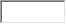/ Homework Answers / Physics / Question The 1 kg standard body has an acceleration of 2.00
Not my Question
Flag Content

# Question : Question The 1 kg standard body has an acceleration of 2.00

Question

The 1 kg standard body has an acceleration of 2.00 m/s2at16 to the positive direction of thexaxis.

(a) What are thexandycomponents of the net force on it?N (xcomponent)

N (ycomponent)

(b) What is the net force in unit-vector notation?

Ni+Nj

(a) What are thexandycomponents of the net force on it?

N (xcomponent)

N (ycomponent)

(b) What is the net force in unit-vector notation?

Ni+Nj

## Solution 5 (1 Ratings )

Solved
Physics 7 Months Ago 4 Views